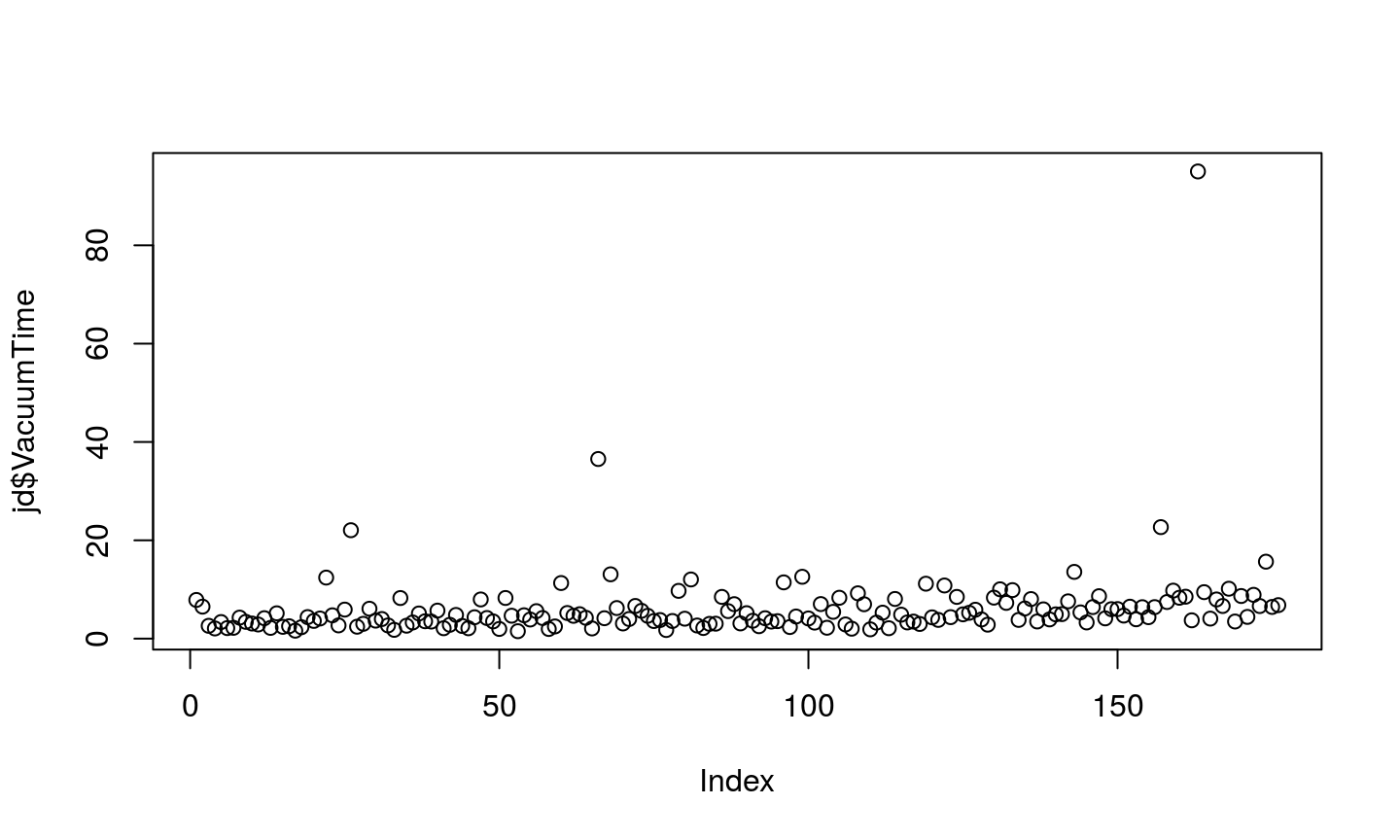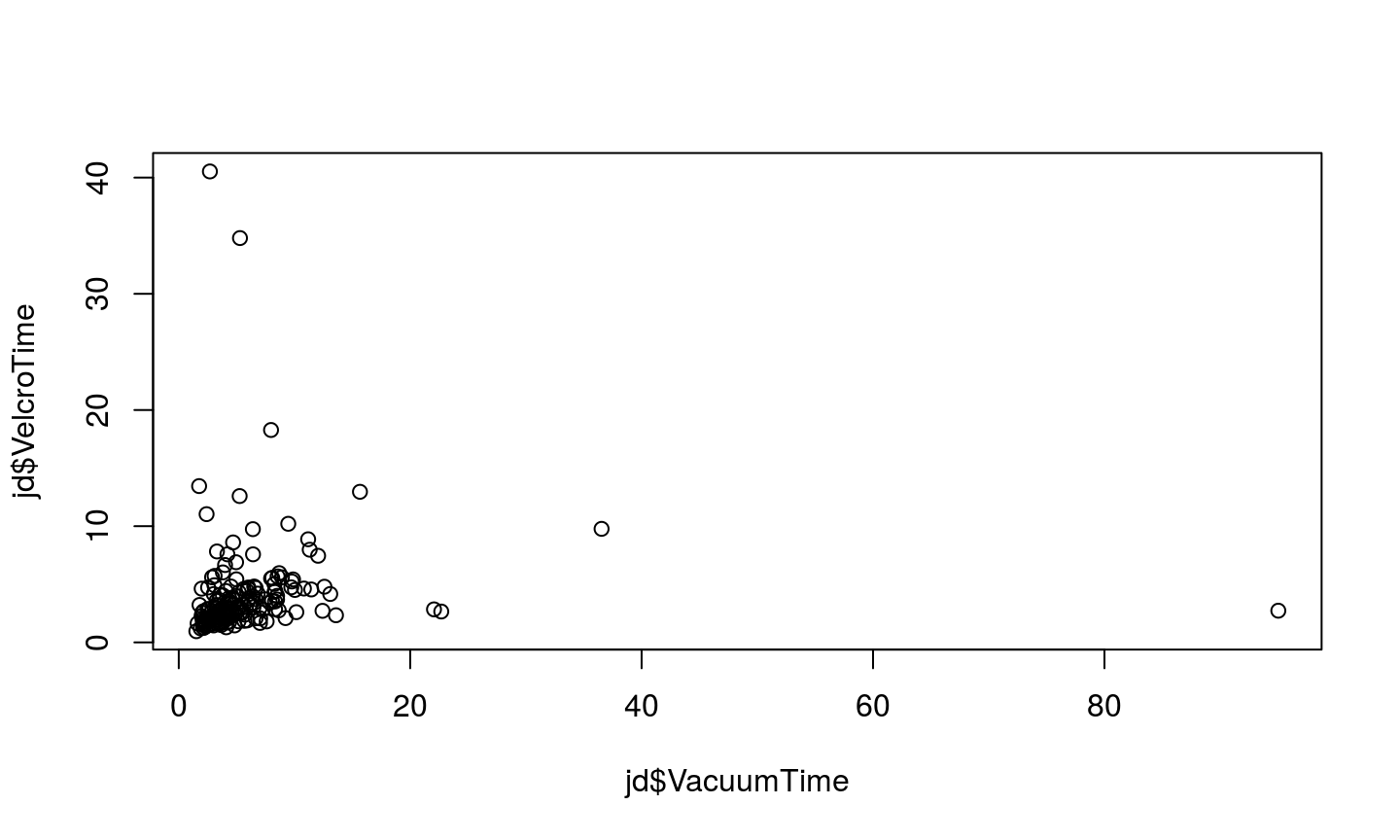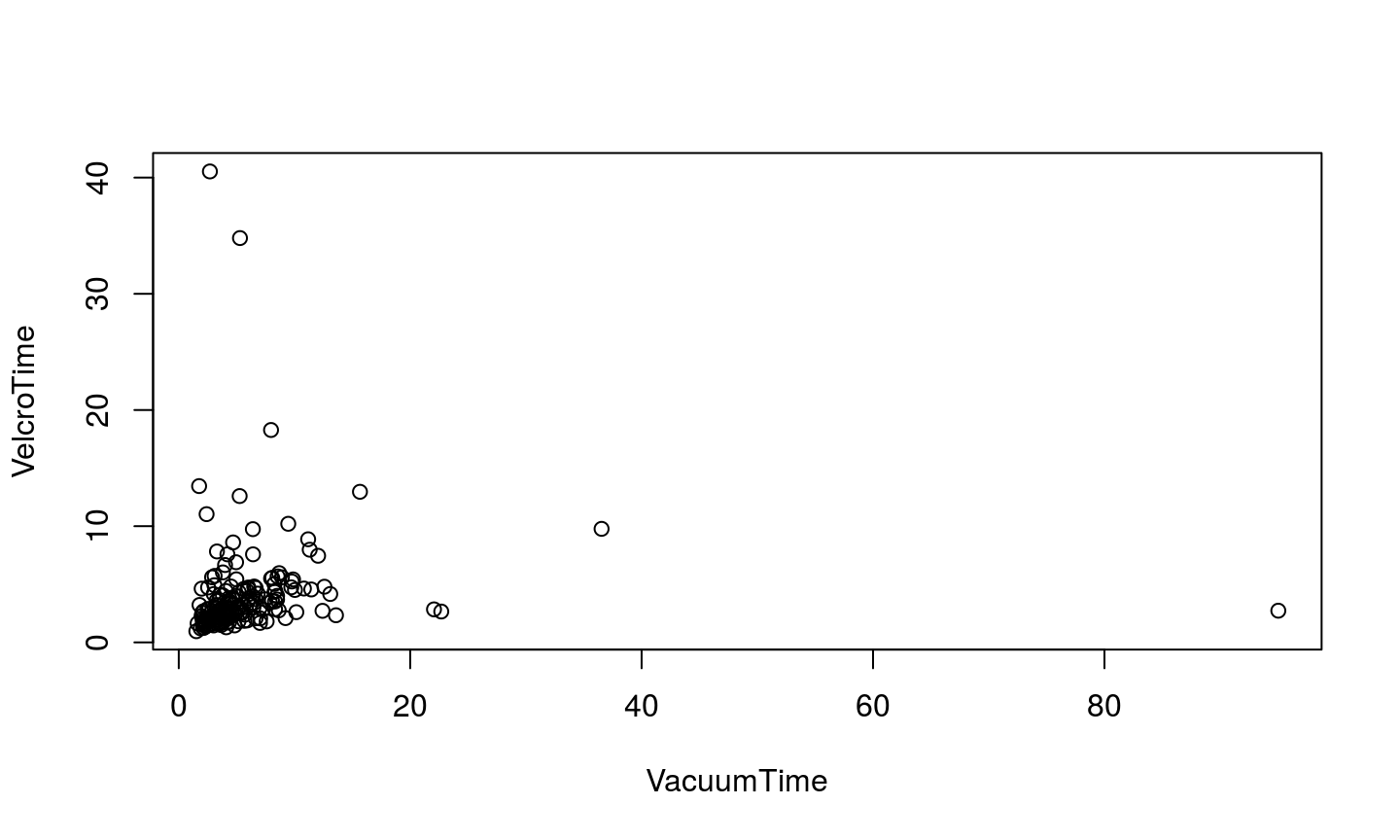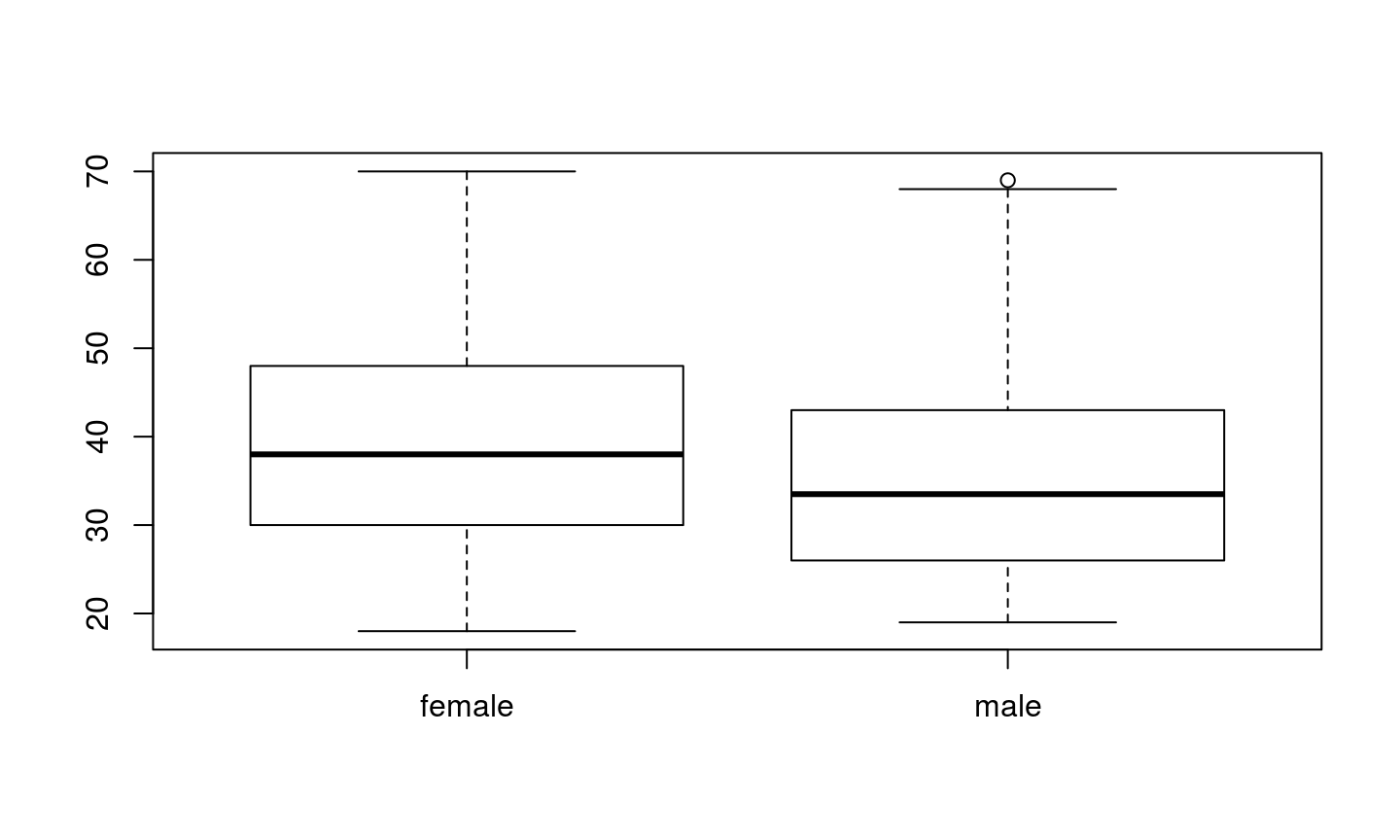Start the exercise

To update your project with the data and .rmd file for this exercise, run:

bio297::start()

Mechanical Turk survey

Download the file johnsonlab.xlsx from the data/ subfolder and take a quick look at it in Excel.

Reading from an Excel file

I've already installed readxl for you, but if I hadn't you could install this package with:

To load the functions in readxl into our current session, we'll use library:

Packages and help pages

At the console you can use:

##  "excel_sheets" "read_excel"

The read_excel function seems simple enough! Let's use it to load data from the first sheet:

Why didn't we have to specify a sheet argument above?

Getting the lay of the land

View(jd)
• The data is tidy: each variable has a column, each observation a row
• Variable names are formatted consistently
• Variable names start with letters and have no spaces

These data are also a nice mix of variable types. We have:

• Continuous data: VacuumTime
• Discrete data: VacuumUnderstanding
• Categorical data: Gender
class(jd$VacuumTime) ##  "numeric" class(jd$VacuumUnderstanding)
##  "numeric"

Now let's check on a categorical variable:

class(jd$Gender) ##  "numeric" The facts about Factors • Use character to hold arbitrary text: for example codon sequences • Use factor to hold true categorical variables (Gender) with defined levels (male, female). gender <- factor( c("male", "female") ) gender ##  male female ## Levels: female male levels(gender) ##  "female" "male" You can index factors just like any other type of vector: gender[ c(1, 1, 2, 2, 1) ] ##  male male female female male ## Levels: female male Using factors to model categorical data We can replace the current numeric column with a factor: jd$Gender <- gender[ jd$Gender ] Check to make sure that worked and verify that you understand why it did! # Enter your code here! Summary statistics You can call summary on an entire data frame: summary(jd) Or just one vector: summary(jd$VacuumTime)
##    Min. 1st Qu.  Median    Mean 3rd Qu.    Max.
##   1.519   3.286   4.384   6.092   6.696  95.030
summary(jd$Gender) ## female male ## 118 58 Basic plotting Just like summary, the plotting functions like plot and boxplot are also a little bit magical in R. We can plot one numeric column: plot(jd$VacuumTime)Or make a scatter plot with two:

plot(jd$VacuumTime, jd$VelcroTime)Formula syntax

So that last scatter plot in formula syntax would be:

plot(VelcroTime ~ VacuumTime, data = jd)boxplot(Age ~ Gender, data = jd)